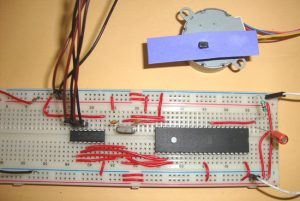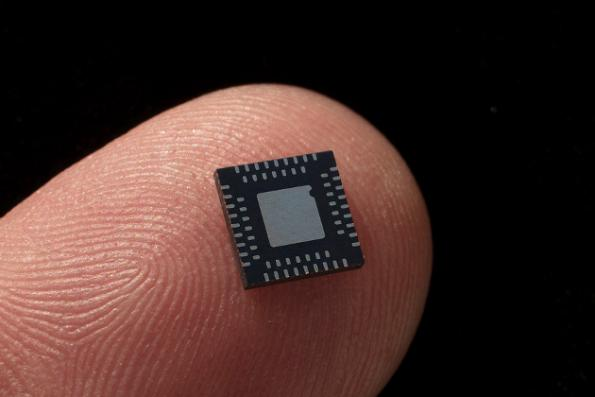# STEPPER MOTOR INTERFACING WITH 8051 MICROCONTROLLER

Stepper motor is brushless DC motor, which can be rotated in small angles, these angles are called steps. Generally stepper motor use 200 steps to complete 360 degree rotation, means its rotate 1.8 degree per step. Stepper motor used in many devices which needs precise rotational movement like robots, antennas, hard drives etc. We can rotate stepper motor to any particular angle by giving it proper instructions.Stepper motors are basically two types: Unipolar and Bipolar. Unipolar stepper motor generally has five or six wire, in which four wires are one end of four stator coils, and other end of the all four coils is tied together which represents fifth wire, this is called common wire (common point). Generally there are two common wire, formed by connecting one end of the two-two coils as shown in below figure. Unipolar stepper motor is very common and popular because of its ease of use.

In Bipolar stepper motor there is just four wires coming out from two sets of coils, means there are no common wire.

Stepper motor is made up of a stator and a rotator. Stator represents the four electromagnet coils which remain stationary around the rotator, and rotator represents permanent magnet which rotates. Whenever the coils energised by applying the current, the electromagnetic field is created, resulting the rotation of rotator (permanent magnet). Coils should be energised in a particular sequence to make the rotator rotate. On the basis of this “sequence” we can divide the working method of Unipolar stepper motor in three modes: Wave drive mode, full step drive mode and half step drive mode.

READ  PIC microcontroller led flasher circuit using PIC16F627A

Wave drive mode: In this mode one coil is energised at a time, all four coil are energised one after another. It produces less torque in compare with Full step drive mode but power consumption is less. Following is the table for producing this mode using microcontroller, means we need to give Logic 1 to the coils in the sequential manner.

Full Drive mode: In this, two coil are energised at the same time producing high torque. Power consumption is higher. We need to give Logic 1 to two coils at the same time, then to the next two coils and so on.

Half Drive mode: In this mode one and two coils are energised alternatively, means firstly one coil is energised then two coils are energised then again one coil is energised then again two, and so on. This is combination of full and wave drive mode, and used to increase the angular rotation of the motor.

### Interfacing Stepper Motor with 8051 Microcontroller

Interfacing with 8051 is very easy we just need to give the 0 and 1 to the four wires of stepper motor according to the above tables depending on which mode we want to run the stepper motor. And rest two wires should be connected to a proper 12v supply (depending on the stepper motor). Here we have used the unipolar stepper motor. We have connected four ends of the coils to the first four pins of port 2 of 8051 through the ULN2003A.

8051 doesn’t provide enough current to drive the coils so we need to use a current driver IC that is ULN2003A. ULN2003A is the array of seven NPN Darlington transistor pairs. Darlington pair is constructed by connecting two bipolar transistors to achieve high current amplification. In ULN2003A, 7 pins are input pins and 7 pins are output pins, two pins are for Vcc (power supply) and Ground. Here we are using four input and four output pins. We can also use L293D IC in place of ULN2003A for current amplification.

You need to find out four coil wires and two common wires very carefully otherwise motor will not rotate. You can find it out by measuring resistance using multimeter, multimeter won’t show any readings between the wires of two phases. Common wire and the other two wire in the same phase should show the same resistance, and the two end points of the two coils in the same phase will show the twice resistance in compared with resistance between common point and one end point.

### Troubleshooting

If your motor is not rotating OR vibrating but not rotating, then you must check the following checklist:

1. First check the circuit connections and code.
2. If the circuit and code is ok, then check that the stepper motor gets proper supply voltage (generally 12v), otherwise it just vibrate but not rotate.
3. If supply is fine, then check the four coil end points which in connected to ULN2003A. First find the two common end points and connect them to 12v, then connect the remaining four wires to ULN2003A and try every possible combination until motor get started. If you wouldn’t connect them in proper order then the motor just vibrate instead of rotating.

Here is the code for Wave step mode and full wave step mode, you can easily calculate the value for PORT P2 for the half wave mode.

#### Current Project / Post can also be found using:

• list the electrical laboratory and submit the report on construction and wofking of stepper motor when it is interfaced with any of mico controller